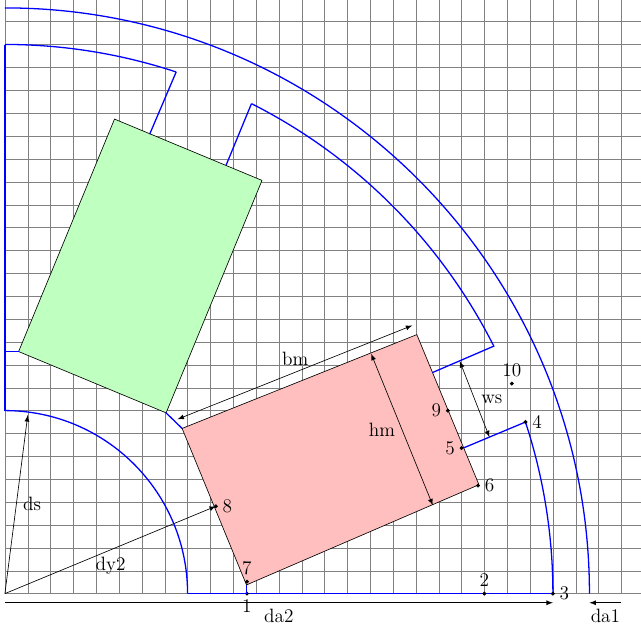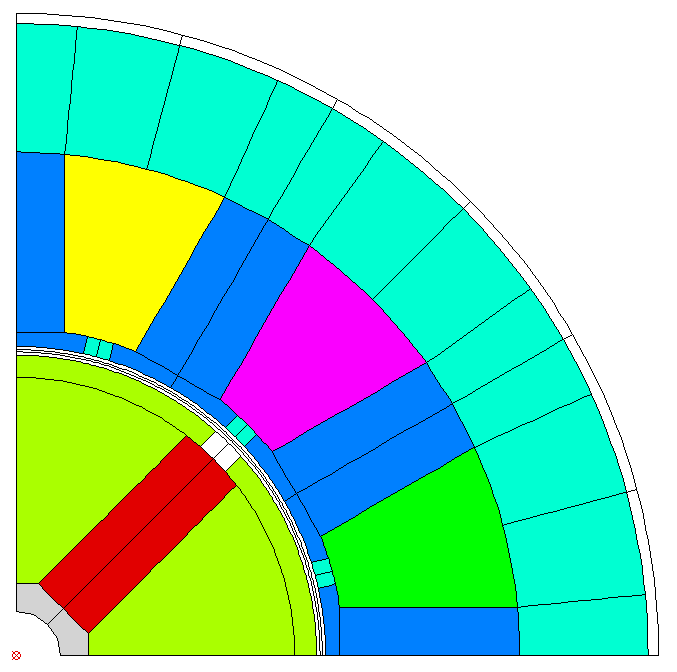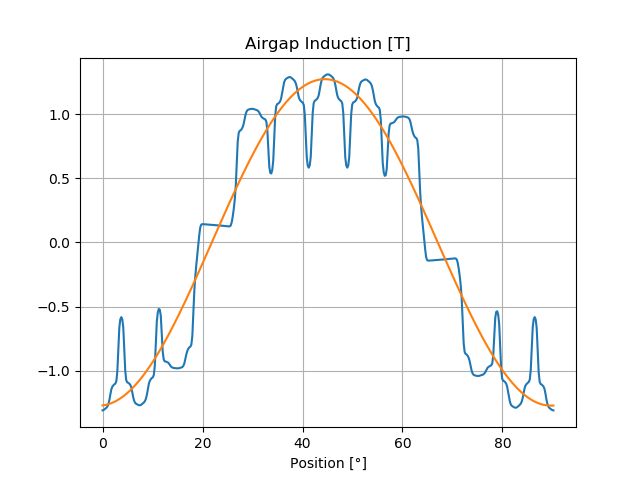# Models¶

The models are dictionaries with the properties of a machine or a calculation.

## Machine¶

Machines have a set of basic parameters, a stator, a magnet and a winding:

Parameter Description Unit
name Name of machine
lfe Lenght of iron m
poles Number of poles
outer_diam Outer diameter (yoke side) m
bore_diam Bore diameter (airgap side) m
inner_diam Inner diameter (yoke) m
airgap airgap width m
external_rotor True, False False
ffactor processing factor for iron losses
dxffile (see Model Creation with DXF)

### Stator¶

Stators have basic parameters and slots:

Parameter Description Default
num_slots Number of Slots Q
num_slots_gen Number of Slots in Model m*Q/gcd(Q, 2p*m)
rlength Relative iron length 1.0
mcvkey_yoke Name of lamination material dummy
mcvkey_teeth Name of lamination material dummy
nodedist Factor for node distance 1.0

Note

if no value for num_slots_gen is given its value is calculated from the the number of slots Q and pole pairs p. (version added 0.0.16)

#### Stator Slots¶

Name Parameter
stator1 slot_rf1, tip_rh1, tip_rh2, tooth_width, slot_width
stator2 slot_t1, slot_t2, slot_t3, slot_depth, slot_width, corner_width
statorRotor3 slot_height, slot_h1, slot_h2, slot_width, slot_r1, slot_r2, wedge_width1, wedge_width2, middle_line, tooth_width, slot_top_sh
stator4 slot_height, slot_h1, slot_h2, slot_h3, slot_h4, slot_width, slot_r1, wedge_width1, wedge_width2, wedge_width3
statorBG yoke_diam_ins slot_h1, slot_h3, slot_width, slot_r1, slot_r2, middle_line, tooth_width, tip_rad, slottooth
<filename> (see User defined Stator Slots with FSL)
dxffile (see User defined Slots with DXF)

Note

All units are metric units.

#### User defined Stator Slots with FSL¶

If a FSL file that includes the definition of stator geometry exists and is readable it can be used for the model creation.

Example with file mystator.fsl:

```machine = dict(
name="Motor",
...
stator=dict(
mcvkey_yoke='dummy',
mcvkey_shaft="dummy",
mystator=dict()
),
...
```

#### User defined Slots with DXF¶

If a DXF file that defines the stator geometry exists and is readable it can be used to create the FSL of the model. All DXF conversion parameters are supported.

Example:

```machine = dict(
name="Motor",
...
stator=dict(
mcvkey_yoke='dummy',
dxffile=dict(
name="mystator.dxf",
position='out',
split=True
)
),
...
```
Parameters Description Default
position ‘in’ or ‘out’
split splits intersecting lines at their intersection-points False
plot creates the plot of the integrated object False

– Note:: The split option is required only if intersecting lines have no common point.

### Windings¶

Name Parameter Default
num_phases number of phases (m)
num_wires number of wires per slot
coil_span coil span
num_layers number of layers
cufilfact Fill factor of copper 0.45
culength rel length of conductor 1.4
slot_indul insulation thickness in slot 0.0

Windings may contain a leakage dict: leak_dist_wind, leak_evol_wind, leak_tooth_wind (version added 0.9.9)

• leak_dist_wind

Name Parameter Unit
endheight End winding height m
m.wiredia Wire diameter m
• leak_evol_wind

Name Parameter Unit
botlevel Level at bottom of evolvents m
toplevel Level at top of evolvents m
endheight End winding height m
m.wiredia Wire diameter m
• leak_tooth_wind

Name Parameter Unit
endheight End winding height m
m.wiredia Wire diameter m

Example:

```windings=dict(
num_phases=3,
num_wires=100,
coil_span=3.0,
num_layers=1,
leak_dist_wind=dict(
endheight=20e-3,  # End winding height [m]
wiredia=1e-3)     # Wire diameter [m]
)
```

### Magnet¶

Magnets have basic parameters and slots:

Parameter Description Default
mcvkey_yoke Name of lamination material dummy
mcvkey_shaft Name of shaft material dummy
material Name of magnet material
nodedist Factor for node distance 1.0

Note

• the mcvkey parameters either reference a filename without extension (Example ‘M330-50A’) which must be found in the directory defined by the parameter magnetizingCurves of the Femag constructor or the name of an entry in the magnetizingCurve object.
• the material parameter references a name of the ‘Magnet Material’_ list.

#### Rotor Slots¶

Name Parameter
magnetSector magn_num, magn_width_pct, magn_height, magn_shape, bridge_height, magn_type, condshaft_r, magn_ori, magn_rfe, bridge_width, magn_len
magnetIron magn_height, magn_width, gap_ma_iron, air_triangle, iron_height, magn_rem, condshaft_r, magn_ori, bridge_height, bridge_width, iron_shape
magnetIron2 magn_height, magn_width, gap_ma_iron, air_triangle, iron_height, magn_rem, condshaft_r, gap_ma_right, gap_ma_left, magn_ori, iron_shape
magnetIron3 magn_height, iron_bfe, gap_ma_iron, air_triangle, iron_height, gap_ma_right, gap_ma_left, condshaft_r, magn_num, magn_ori, iron_shape
magnetIron4 magn_height, magn_width, gap_ma_iron, iron_shape, air_space_h, iron_bfe, magn_di_ra, corner_r, air_sp_ori, magn_ori, magn_num
magnetIron5 magn_height, magn_width, gap_ma_iron, iron_bfe, air_space_h, corner_r, air_sp_ori, magn_num, iron_shape, air_space_b, magn_di_ra
magnetIronV magn_height, magn_width, magn_angle, magn_num, iron_hs, iron_height, iron_shape, air_triangle, gap_ma_iron, magn_rem, condshaft_r
magnetFC2 yoke_height, iron_h1, iron_h2, iron_b, magn_width, magn_height, iron_bfe, iron_bfo, iron_shape, iron_hp, magn_num
<filename> see User defined Magnet Slots with FSL
dxffile see User defined Slots with DXF

Example:

```machine = dict(
name="PM 130 L4",
lfe=0.1,
poles=4,
outer_diam=0.13,
bore_diam=0.07,
inner_diam=0.015,
airgap=0.001,

stator=dict(
num_slots=12,
num_slots_gen=3,
mcvkey_yoke="dummy",
rlength=1.0,
stator1=dict(
slot_rf1=0.057,
tip_rh1=0.037,
tip_rh2=0.037,
tooth_width=0.009,
slot_width=0.003)
),

magnet=dict(
mcvkey_shaft="dummy",
mcvkey_yoke="dummy",
magnetSector=dict (
magn_num=1,
magn_width_pct=0.8,
magn_height=0.004,
magn_shape=0.0,
bridge_height=0.0,
magn_type=1,
condshaft_r=0.02,
magn_ori=2,
magn_rfe=0.0,
bridge_width=0.0,
magn_len=1.0 )
),

windings=dict(
num_phases=3,
num_wires=100,
coil_span=3.0,
num_layers=1)
)
```

#### User defined Magnet Slots with FSL¶

Example

If a FSL file that creates the magnet geometry exists and is readable it can be used for the model creation as an empty dict:

```machine = dict(
name="Motor",
...
magnet=dict(
mcvkey_yoke='dummy',
mcvkey_shaft="dummy",
myrotor=dict()
),
...
```

#### User defined Slots with DXF¶

If a DXF file that defines the magnet geometry exists and is readable it can be used to create the FSL for the model.

Example:

```machine = dict(
name="Motor",
...
magnet=dict(
mcvkey_yoke='dummy',
mcvkey_shaft="dummy",
dxffile=dict(
name='mymagnet.dxf',
position='in',
split=True
)
),
...
```
Parameters Description Default
position ‘in’ or ‘out’
split splits intersecting lines at their intersection points False
plot creates the plot of the integrated object False

– Note:: The split option is required only if intersecting lines have no common point.

## Magnetizing Curve¶

The MagnetizingCurve is a container of magnetizing curves (eg. lamination or PM material) that can be referenced by the model mcvkey attributes. It can either point to a directory of MC/MCV-File or hold a list of magnet curves which are identified by name.

Each magnetizing curve is described by the following properties

Attribute Description Unit Default
name Identifier of this curve
ctype Type of curve   1
desc Description
curve List of dictionaries with bi (list of induction values) hi (List of field strength values) and angle which can be missing in case of 1 curve T, A/m, deg
ch hysteresis loss factor   0
cw eddy current loss factor   0
ch_freq hysteresis exponent   0
cw_freq eddy-current exponent   0
b_coeff induction loss exponent   0
Bo reference induction T 1.5
fo reference frequency Hz 50
fillfac iron fill factor   1
bsat saturation induction T 2.15
rho specific weight kg/dm3 7.65

The loss factors and exponents are used in the Jordan loss calculation formula:

(cw*(f/fo)**cw_freq + ch*(f/fo)**ch_freq)*(B/Bo)**b_coeff

The Reader object which is included in the mcv module can be used to read MCV/MC files.

Permeability and polarisation calculation example:

```MUE0 = 4e-7*math.pi

r = mcv.get_results()

bh = [(bi, hi)
for bi, hi in zip(r['curve']['bi'],
r['curve']['hi']) if bi > 0 and hi > 0]

ji = [b-MUE0*h for b, h in bh]
muer = [bx/hx/MUE0 for bx, hx in bh]
```

Using a magnetizingcurve to write a mcv file:

```mcvData = dict(curve=[ dict(
bi=[0.0, 0.09, 0.179, 0.267, 0.358,
0.45, 0.543, 0.6334, 0.727,
0.819, 0.9142, 1.0142, 1.102,
1.196, 1.314, 1.3845, 1.433,
1.576, 1.677, 1.745, 1.787,
1.81, 1.825, 1.836],

hi=[0.0, 22.16, 31.07, 37.25, 43.174,
49.54, 56.96, 66.11, 78.291,
95, 120.64, 164.6, 259.36,
565.86, 1650.26, 3631.12, 5000, 10000,
15000, 20000, 25000, 30000, 35000, 40000]
)],
name='m270-35a',
desc=u"Demo Steel",
ch=4.0,
cw_freq=2.0,
cw=1.68)

mcv = femagtools.mcv.MagnetizingCurve(mcvData)

mcv.writefile('m270-35a')
```Note

if the curve data is used in a stator or magnet slot model there is no need to create the file explicitly. Femagtools will take care of that during the model creation.

## Magnet Material¶

list of dict objects each having a unique name (or id) and a set of parameters that describe the magnet properties.

Parameter Description Default Unit
name Name of magnet material
mcvkey name of nonlinear B(H) curve
orient Magnetizing orientation mpolaniso
rlen Relative length 1.0
remanenc Remanence Induction Br   T
relperm Relative Permeability
spmaweight Specific Mass 7500 kg/m³
temcoefbr Temperature Coefficient of Br -0.001 T/K
temcoefhc Temperature Coefficient of Hc -0.001 A/m/K
magntemp Magnet Temperature 20 °C
magncond Electr. Conductivity 625000 S/m
magnwidth Magnet width 0.0 m
magnlength Magnet length in z direction 0.0 m

Note

• name must be unique within list. It may be used as reference in the magnet model of the machine.

Example:

```magnets = [dict(name='MX-333', remanenc=1.2, relperm=1.05)]
```
• mcvkey is used for material that have a non-linear BH curve.

• the key orient describes the field orientation (mcartiso, mpoliso, martaniso, mpolaniso)

• rlen defines the relative length

Example:

```magnets=[dict(name='BH53M', mcvkey='BH53M',
orient='mcartiso', rlen=1.0)]
```
• The mcvkey can either reference a file or an entry in the magnetizing curve dict.

## User Specified Magnet Geometries¶

Magnet geometries not covered by the built in models can be specified by fsl code. Here is a example:Example of a user specified geometry (created with TikZ)

The steps are:

1. Create a diagram of the geometry and decide about the parameters to be used.
2. Create a fsl mako template file with the chosen parameters as placeholders using the Mako syntax with \${}.
3. Include this filename in the magnet model within the section using the basename of the mako file and set the parameter values.

The following values are defined globally and can be referenced in the template:

Name Description Unit
mcvkey_yoke lamination material of yoke
mcvkey_shaft material of shaft
m.remanenc Remanence Induction T
m.relperm Rel. Permeability of PM
m.num_poles Number of poles
m.npols_gen Number of poles in model
agndst Node distance in airgap m
dy1 Diameter of stator yoke m
da1 Diameter of stator bore m
da2 Diameter of rotor m
dy2 Diameter of rotor yoke m

Example FSL Template file ‘’spoke.mako’’ using 4 parameters:

```-- Model parameters

ds = \${model['shaft_diam']}*1e3
hm = \${model['magn_height']}*1e3
bm = \${model['magn_width']}*1e3
ws = \${model['slot_width']}*1e3

-- calculate slot height and pole pitch
hs = (da2-dy2)/2 - bm
taup = math.pi/m.num_poles

x = {}
y = {}

-- characteristic points of model

ar = math.sqrt(dy2^2+hm^2)/2
x = ar*math.cos(taup - math.atan2(hm/2, dy2/2))
y = 0
x,y = pr2c(dy2/2+bm, 0)
x,y = pr2c(da2/2, 0)
x,y = pr2c(da2/2, taup - math.atan2(ws/2,(da2/2)))
x,y = pr2c(da2/2-hs, taup - math.atan2(ws/2,(da2/2 - hs)))
x,y = pr2c(da2/2-hs, taup - math.atan2(hm/2, da2/2-hs))
x,y = pr2c(ar, taup - math.atan2(hm/2, dy2/2))
x,y = pr2c(dy2/2, taup)
x,y = pr2c(da2/2 - hs, taup)
x,y = pr2c(da2/2, taup)
```

Magnet definition in Python file with included mako file (eg in this case ‘’spoke’‘) and the parameter definition:

```..
magnet=dict(
mcvkey_yoke="dummy",
spoke=dict(
magn_height=0.008,
shaft_diam=0.01,
slot_width=0.004,
magn_width=0.024
)
..
```

The resulting model:Note

Starting with Release 0.4.4 the syntax has changed but backward compatibility is fully supported.

the complete example can be found in stator1-spoke.py and spokefml.mako in the example directory on github.

## Calculation¶

Cogging (cogg_calc)

Parameter Description Default Unit
speed Speed   1/s
skew_angle Skewing angle 0 deg
num_skew_steps Number of skew steps 0
magn_temp Magnet Temperature   °C
num_move_steps Number of move steps
num_par_wdgs Number of parallel windings 1
eval_force Evaluate force 0

Example:

```operatingConditions = dict(
calculationMode="cogg_fast",
magn_temp=60.0,
num_move_steps=49,
speed=50.0)
```

PM/Rel Machine Simulation (pm_sym_fast)

Parameter Description Default Unit
speed Speed   1/s
skew_angle Skewing angle 0 deg
num_skew_steps Number of skew steps 0
magn_temp Magnet Temperature   °C
wind_temp Winding Temperature 20 °C
num_move_steps Number of move steps 49
num_par_wdgs Number of parallel windings 1
eval_force Evaluate force 0
current Phase current   A (RMS)
angl_i_up Angle I vs. Up 0 deg
optim_i_up Optimize Current 0
phi_start Start angle of rotation 0 deg
range_phi Rotation angle 360/p deg
explicit_mode Deactivate rotation correction 0
plots Create plots []
airgap_induc calculate airgap induction False

Note

plots is a list of field_lines or color_gradation plots to be created after the calculation. Possible values ‘field-lines’, ‘Babs’, ‘Br’, ‘Bx’, ‘By’, ‘Br’, ‘Bt’, ‘Habs’, ‘Hx’, ‘Hy’, ‘Hr’, ‘Ht’ ‘demag’, ‘ecurr’, ‘ecloss’, ‘relperm’, ‘Wm’, ‘Bdev’, ‘Vpot’. (See http://script.profemag.ch/ColorGrad.html) added in version 0.0.16. The value types can be simple strings or list with name and min/max range.

Example:

```operatingConditions = dict(
calculationMode="pm_sym_fast",
wind_temp=60.0,
magn_temp=60.0,
current=50.0,
speed=50.0,
plots=['field_lines', ['Babs', 0.0, 2.5]])
```

Note

If airgap_induc is True the induction in the airgap is calculated after the simulation returns. The values can be read with the method read_airgap_induc() of call Femag.

Parameter Description Unit
Baml Amplitude of base harmonic T
phi0 Phase angle of base harmonic rad
pos Position ° or mm
B sampled values T
B_fft Values of base harmonic TLd-Lq Identification (ld_lq_fast)

Parameter Description Default Unit
speed Speed   1/s
skew_angle Skewing angle 0 deg
num_skew_steps Number of skew steps 0
magn_temp Magnet Temperature   °C
num_move_steps Number of move steps
num_par_wdgs Number of parallel windings 1
eval_force Evaluate force 0
i1_max Max. phase current   A (RMS)
beta_min Min. Beta angle   deg
beta_max Max. beta angle   deg
num_cur_steps Number of current steps
num_beta_steps Number of beta steps

Example:

```feapars = dict(
num_move_steps=25,
calculationMode="ld_lq_fast",
magn_temp=60.0,
i1_max=150.0,
beta_max=0.0,
beta_min=-60.0,
num_cur_steps=3,
num_beta_steps"=4,
speed=50.0)
```

Psid-Psiq Identification (psd_psq_fast)

Parameter Description Default Unit
speed Speed   1/s
skew_angle Skewing angle 0 deg
num_skew_steps Number of skew steps 0
magn_temp Magnet Temperature   °C
num_move_steps Number of move steps
num_par_wdgs Number of parallel windings 1
eval_force Evaluate force 0
maxid Max. Amplitude Id current   A
minid Min. Amplitude Id current   A
maxiq Max. Amplitude Iq current   A
miniq Min. Amplitude Iq current   A
delta_id Delta of Id current steps   A
delta_iq Delta of Iq current steps   A

Example:

```feapars = dict(
num_move_steps=25,
calculationMode="psd_psq_fast",
magn_temp=60.0,
maxid=0.0,
minid=-150.0,
maxiq=150.0
miniq=0.0,
delta_id=50.0,
delta_iq=50.0,
speed=50.0)
```

PM/Rel Machine Simulation (pm_sym_fast)

Parameter Description Default Unit
speed Speed   1/s
skew_angle Skewing angle 0 deg
num_skew_steps Number of skew steps 0
magn_temp Magnet Temperature   °C
wind_temp Winding Temperature 20 °C
num_move_steps Number of move steps 49
num_par_wdgs Number of parallel windings 1
current Phase current   A (RMS)
angl_i_up Angle I vs. Up 0 deg

Example:

```operatingConditions = dict(
calculationMode="torq_calc",
wind_temp=60.0,
magn_temp=60.0,
current=50.0,
angl_i_up=0.0,
speed=50.0)
```

### Calculation with existing model¶

FE calculations can be executed for existing models also. Since Femag Rel 8.3 there is no need to fully specify the machine model:

```machine = "PM 270 L8"

workdir = os.path.join(
os.path.expanduser('~'), 'femag')

femag = femagtools.Femag(workdir)

operatingConditions = dict(
angl_i_up=-38.7,
calculationMode="pm_sym_fast",
magn_temp=60.0,
num_move_steps=25,
speed=50.0,
wind_temp=60.0,
current=108.0)

r = femag(machine,
operatingConditions)
```

For older FEMAG versions the minimal data is:

```machine = dict(
name="PM 130 L4",
lfe=0.1,
poles=4,
outer_diam=0.13,
bore_diam=0.07,
airgap=0.001,

stator=dict(
num_slots=12,
num_slots_gen=3,
mcvkey_yoke="dummy"
)
)
```

## Model Creation with DXF¶

The goal of the dxfsl modules is to create a complete FE model for rotating PM and Reluctance Machines on the basis of a DXF file with as little restrictions as possible. This has the consequence that symmetries, subregions, magnets, windings, boundary conditions need to be identified.

The procedure is as follows:

1. Read the DXF file and create a graph object with nodes and edges.
2. Identify the areas stator, rotor and airgap and their symmetry axis.
3. Add auxiliary lines if required by the meshing process.
4. Convert the graph object into FSL code including the identified subregions.

For monitoring and trouble-shooting purposes it is possible to create plots that display the intermediate results.

Example with a single dxf file motor.dxf:

```machine = dict(
name="Motor",
lfe=0.001,

dxffile=dict(
name='motor.dxf'
),
stator=dict(
mcvkey_yoke='dummy',
mcvkey_shaft="dummy"
),
magnet=dict(
mcvkey_yoke="dummy",
mcvkey_shaft="dummy"
),
...
```

Note

The parameters poles, outer_diam, bore_diam and airgap as well as num_slots and num_slots_gen will be set automatically

Example with two separate dxf files for stator and rotor:

```machine = dict(
name="Motor",
lfe=0.001,

stator=dict(
mcvkey_yoke='dummy',
dxffile=dict(
name='mystator.dxf',
position='out',
split=True
)
),
magnet=dict(
mcvkey_yoke="dummy",
mcvkey_shaft="dummy",
dxffile=dict(
name='myrotor.dxf',
position='in',
split=True
)
),
...
```